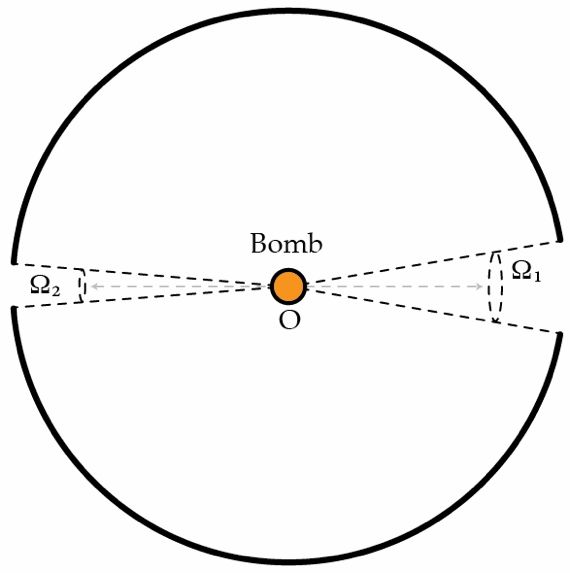# Shrapnel

A bomb of mass $M_{B}$ is located at a center of a spherical shell of mass $M_{s}$ that has two diametrically opposed orifices subtending solid angles $\Omega_{1}=\pi/2$ and $\Omega_{2}=\pi/3$. The bomb explodes, isotropically, into many fragments of equal mass and velocity $v=320~\text{m}/\text{s}$. If $M_{s}=M_{B}$, determine the final velocity of the shell in m/s. Assume that the collisions between the fragments and the shell are perfectly inelastic and ignore gravity.×

Problem Loading...

Note Loading...

Set Loading...